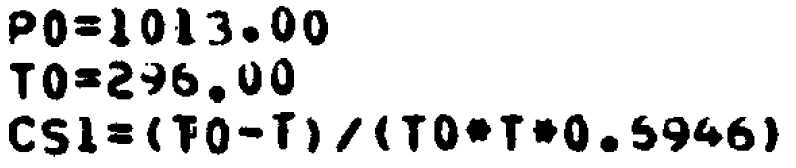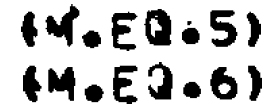### Science Facts HITRAN Equations

I have written a program to plot the "simulated" (reconstructed) spectra of gases based on data provided in the HITRAN database .. simple.

The only problem is that the necessary equations are not provided.

That's right .. there is all this great data .. and no equations.

Oh, they say - See the references. .. what a joke.

You know, maybe this stuff is in some textbook I don't have .. but !!

Therefore, I tried to use other references I found online. Just to make this fun, those references don't agree. Some have errors, others appear to be radically different. We're not talking "improvements" .. these are totally different equations.

### Simple band broadening

This is provided as just an example. There are other problems using the data.

When the temperature and pressure of a gas change, the widths of the spectral lines change. There are no equations that describe this .. however, the Lorentz approximation is close enough for most uses. (The simple fact of using a computer to make the computations may introduce as much error / uncertainty do to sampling and round off problems.)

I used a lot of references, but finally decided to base my code on Appendix B of AFCRL Atmospheric Absorption Line Parameters Compilation (1973), mainly because of problems trying to handle the lower state energy field in the HITRAN data. Note that part of this reference (a pdf file) is an image and part is searchable text. As a result, if you can't find something by searching, then you must read the entire document. (This is a primary source - and it can not be searched.) Also, since some parts of the document are a photograph of poorly printed and broken characters, it is frequently a challenge to determine exactly what is being said. With regular text (prose), it is not too bad. But with equations and computer code (where single character errors are important), well .. it is more difficult than you would think.

The important equations for this example are (in Pascal)

 ``` CS1 := (T0 - Temperature)/(T0 * Temperature * 0.69503563); // inside a loop dp.Adjusted_Intensity := dp.Line_Intensity * CS2 * exp(-dp.lower_state_energy * CS1) ; ```
In the provided Fortran code (page 75, Appendix B, pdf page 81)
 ```CS1 = (T0-T)/(T0*T*0.5946) S(I)=S(I)*CS2(M)*EXP(-EDP(I)*CS1) ```
The first thing to note is the constant
 ```0.5946 ```
Description Image from pdf file
Normal SizeMagnified
422%Another 5 and 6As shown above, this part of the pdf is an image. On careful examination, I have determined that the "5" is actually a "6". This was particularly difficult because the lower part looked exactly like a "5" (open on the left). In general, if that part of the other 6's on the page was missing, they would have had significantly thinner lines in other areas (as seen in the lower left of the last "6" in 6946). On the other hand, the angle on the upper part of a "5" is almost square and that part of this character is exactly like a "6". At any rate, the only way that this can be a "6" is if it was printed using a different piece of type from the other 6's. In other words, this must have been printed on a drum printer (as opposed to a chain printer or a computer controlled type writer).

So I interpreted the code as

 ```CS1 = (T0-T)/(T0*T*0.6946) ```

Well the references (plural) clearly state that the constant is k/hc. (In the box below, values and units are from Google.)

 ```k = Boltzmann constant = 1.3806503 × 10-23 m2 kg s-2 K-1 h = Planck's constant = 6.626068 × 10-34 m2 kg / s c = Speed of light = 299 792 458 m / s k/hc = 69.50356804 m-1 K-1 k/hc = 0.6946 used in the supplied program ```
Well .. that's interesting. they are almost the same .. except for a factor of 100. As you can see in the examples above, CS1 is computed in such a way that the "K" dimension disappears .. but not the distance. Eventually, CS1 is multiplied by EDP(I) (a value read from the supplied data) before being used as an exponent (which MUST have no dimensions).

EDP(I) is the "lower state energy" and provided per spectral line in the HITRAN database. A quick check of the provided data formats did not revel what the appropriate dimensions should be. (They most definitely should have been there.) A "search" of the reference also provided NO information.

As I noted above, the reference pdf file is partly a scanned document and partly text. As a result, my "search" (using the pdf tools) found nothing because they can not search the "scanned" parts of the document. When I took time to actually read the document, I found the necessary information on page 2 (page 8 of the pdf).

 ```the energy of the lower state, E"(cm-1) ```
and on page 7 (page 13 of the pdf).
 ```E" = energy of the lower state expressed in wavenumbers. ```
Therefore, multiplying CS1 (in centimeters) times the "energy" (I would have assumed watts) causes the units to cancel producing the needed dimensionless number. This also explained the factor of 100 - it was needed to convert meters to centimeters.

At this point, it was time to compare their value with mine.

 ``` mine = 0.6950356804 theirs = 0.6946 ```
When reading old code, I frequently find small differences like this. Supposedly, it is because we now have better (more accurate) values for the base constants. Perhaps.

Well .. that appears to be a 0.08% error .. close enough for this. (Actually 0.06% to 0.09% depending on how it is computed. Yes .. there ARE different ways. Compare the inverse. Compare after computing EXP(-EDP(I)*CS1).)

Well .. there is more. In the text of the document, it shows (page 3, pdf 9, equation 3)

 ```1.439 E" (T-Ts)/(T * Ts) ```
and
 ```1/1.439 = 0.694927033 -> 0.6949 ```
So .. it appear that their code uses a different constant than the one in the text.

Note that I found this only after I had questions about where a constant came from .. particularly why there was a difference of 100 that I could not explain.

Needless to say, without the example code, I would have missed all of this.

So .. be warned - the equations I have used might be wrong!

I have no way to know. All I can say is that my plots look "reasonable". Oh, and that I have spent - a lot of time - trying to verify the equations I am using. But this is the best I can do without additional help. (hint, hint)

Bottom line - without the provided (and almost unreadable) code in the oldest of the HITRAN references (1973), I would have never gotten a program to work.

Author: Robert Clemenzi